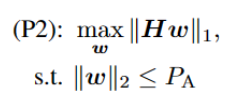# Disciplined convex programming error: Cannot maximize a(n) convex expression

Here is my problem:where H is a N×M complex matrix, each element of H has an amplitude of 0 ~ 1 and a phase of 0 ~ 2π. w is a M ×1 complex variable. PA denotes power (I set PA to 10 in the simulation).
Here is my code:
cvx_begin sdp
variable w(M,1) complex;
maximize (norm(H * w,1))
subject to
norm(w,2) <= PA;
cvx_end
when I run my code, there is a problem: Disciplined convex programming error: Cannot maximize a(n) convex expression. How can I solve the problem?

Because it is non-convex, you can solve it with something other than CVX. For instance, YALMIP.